Question

# Minnie's Mineral Springs, a single-price monopoly, faces the market demand schedule below.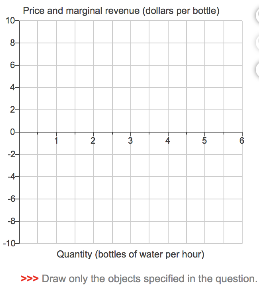Minnie's Mineral Springs, a single-price monopoly, faces the market demand schedule below.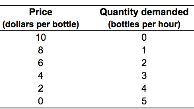Draw a point to show the quantity demanded when

1) the price is \$10. Label it 1.

2) the price is \$2. Label it 2.

Draw the market demand curve through these points and extend it to the x-axis. Label it D.

Draw a point to show marginal revenue when

1) the price falls from \$10 to \$8. Label it 3.

2) the price falls from \$2 to 50. Label it 4.

Draw the marginal revenue curve through these points. Label it MR.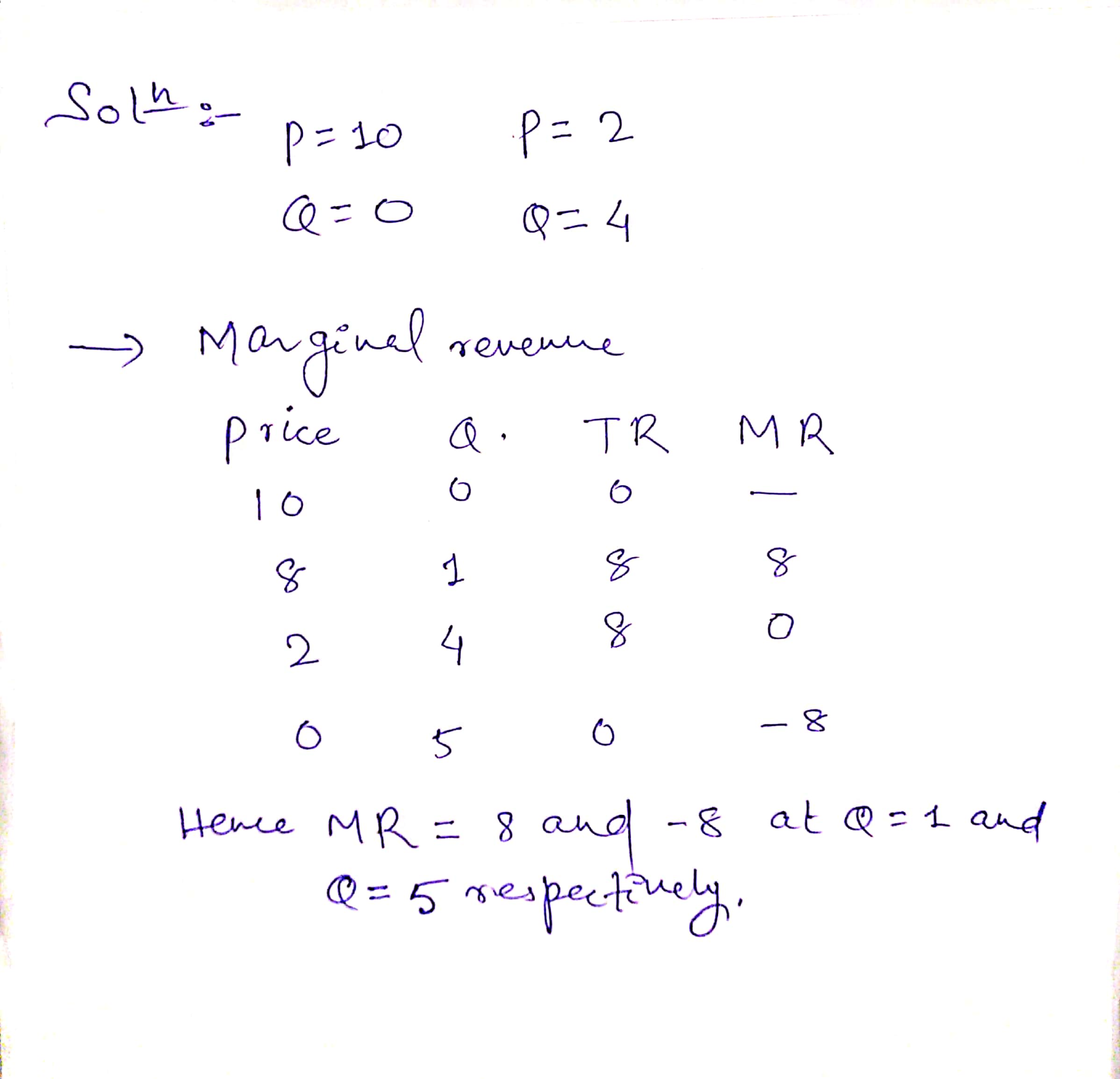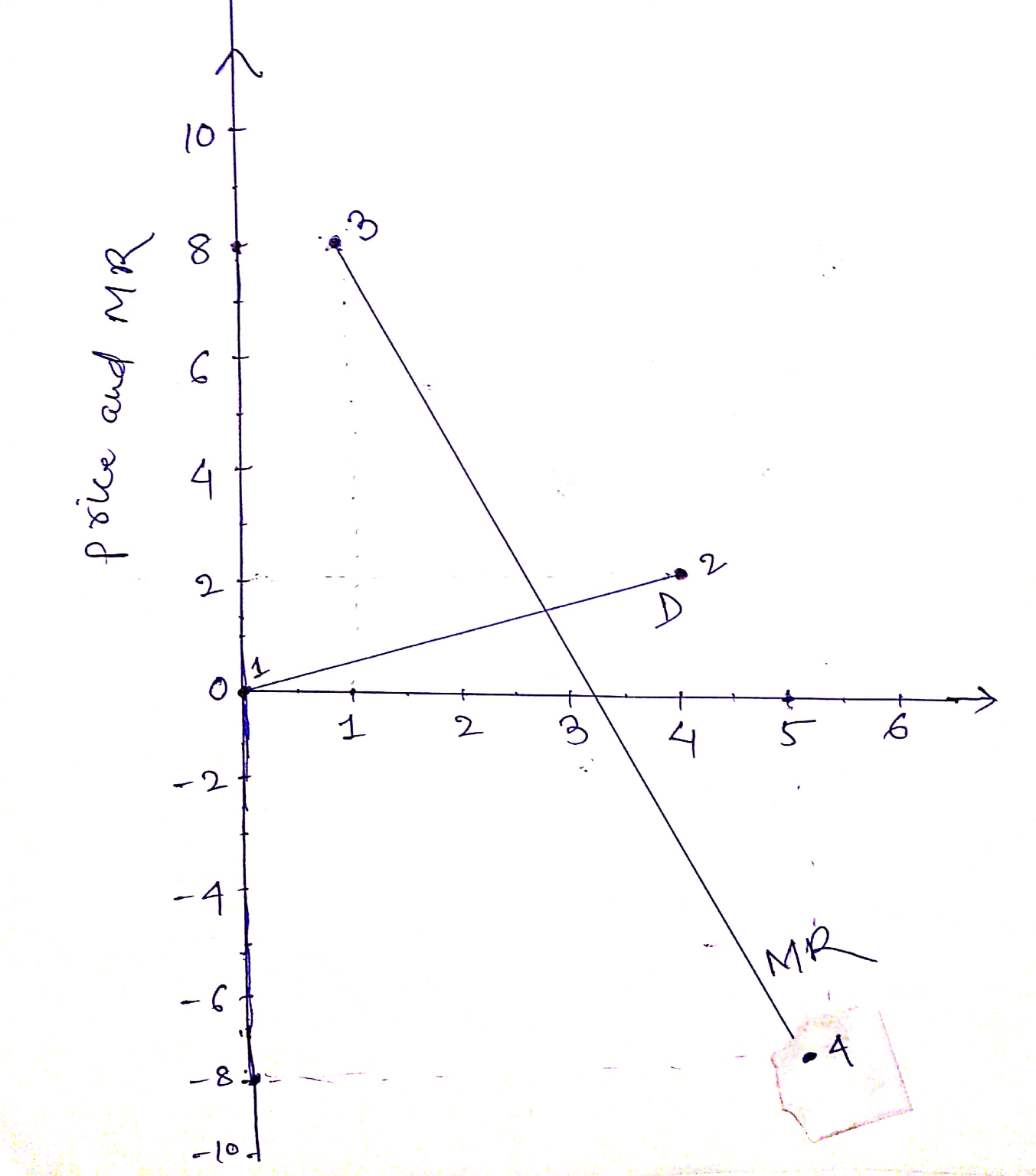#### Earn Coins

Coins can be redeemed for fabulous gifts.

Similar Homework Help Questions
• ### Hot Air Balloon Rides, is a single-price monopoly. The table gives Hot Air's demand schedule. Quantity...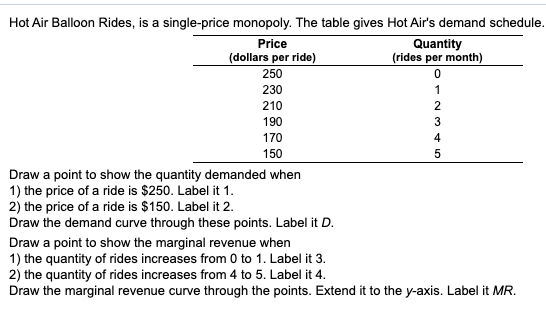Hot Air Balloon Rides, is a single-price monopoly. The table gives Hot Air's demand schedule. Quantity (rides per month) Price (dollars per ride) 250 230 210 190 170 150 Draw a point to show the quantity demanded when 1) the price of a ride is \$250. Label it 1. 2) the price of a ride is \$150. Label it 2. Draw the demand curve through these points. Label it D. Draw a point to show the marginal revenue when 1)...

• ### Fogg's Tall Ship Cruises is a single-price monopoly. The table gives Fogg's demand schedule nd marginal...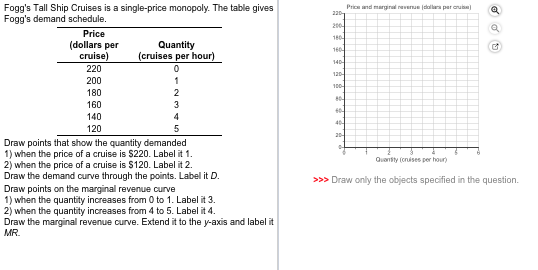Fogg's Tall Ship Cruises is a single-price monopoly. The table gives Fogg's demand schedule nd marginal naveue Price (dollars per Quantity cruise (cruises per hour) 220 200 180 160 140 120 Draw points that show the quantity demanded 1) when the price of a cruise is S220. Label it 1 2) when the price of a cruise is S120. Label it 2 Draw the demand curve through the points. Label it D. Draw points on the marginal revenue curve 1)...

• ### This Quiz: 10 pts possib Price and marginal revenue (dollars per rosebush) 14- 12- 2 10-...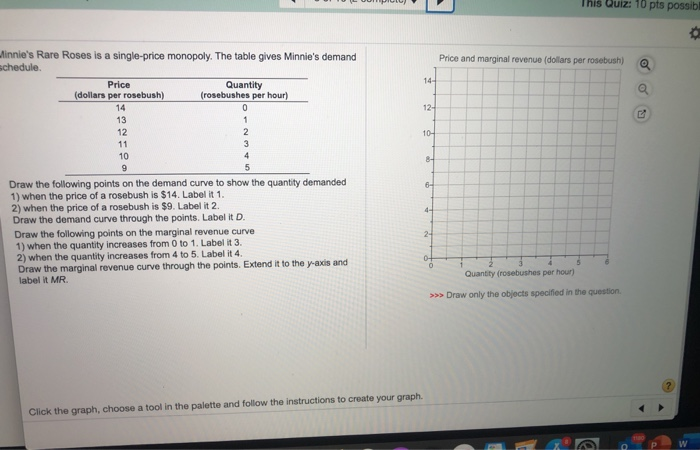This Quiz: 10 pts possib Price and marginal revenue (dollars per rosebush) 14- 12- 2 10- 8- winnie's Rare Roses is a single-price monopoly. The table gives Minnie's demand schedule Price Quantity (dollars per rosebush) (rosebushes per hour) 14 0 13 12 11 3 10 4 9 5 Draw the following points on the demand curve to show the quantity demanded 1) when the price of a rosebush is \$14. Label it 1. 2) when the price of a rosebush...

• ### A monopoly firm faces the following demand curve: P = 25-2.5 QD. 1)Create the demand schedule...

A monopoly firm faces the following demand curve: P = 25-2.5 QD. 1)Create the demand schedule for the firm by increasing quantity demanded in increments of one unit. 2)Produce a table with the total revenue and marginal revenue for the output levels in increments of one unit. 3)If the firm’s marginal cost is constant at \$12.50 per unit, what is the profit maximizing output and price? 4)What is the efficient quantity and price? 5)What is the value of the deadweight...

• ### A monopoly faces the demand curve P = 12 - 1.0Q,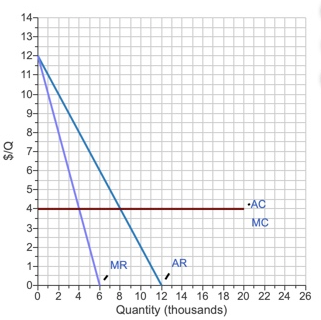A monopoly faces the demand curve P = 12 - 1.0Q, where P is measured in dollars per unit and Q in thousands of units. The monopolist has a constant average cost of \$4.00 per unit. Draw the average and marginal revenue curves and the average and marginal cost curves. 1.) Using the line drawing tool, draw the average revenue curve and label it 'AR'.  2.) Using the line drawing tool, draw the marginal revenue curve and label it 'MR'.  3.) Using the line drawing tool,...

• ### Sam's Mineral Springs is a single-price monopoly. Price Quantity bottles per hour) Total cost The table shows the d...

Sam's Mineral Springs is a single-price monopoly. Price Quantity bottles per hour) Total cost The table shows the demand schedule for Sam's Mineral Springs (columns 1 and 2) and the firm's total cost schedule (columns 2 and 3). (dollars per bottle) (dollars per hour) 15 Suppose Sam's is hit with a conservation tax of \$17 an hour. 14 13 What is Sam's new profit-maximizing output, price, and economic profit? 12 19 When Sam's produces its new profit-maximizing output, the number...

• ### Total cost (dollars r hour Quantity (bottles r hour Price Sam's Mineral Springs is a single-price monopoly (dollars...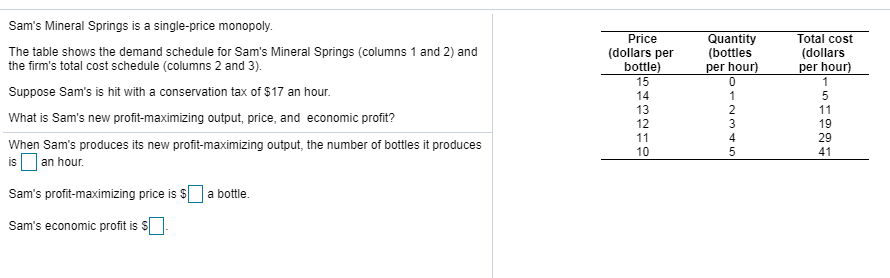Total cost (dollars r hour Quantity (bottles r hour Price Sam's Mineral Springs is a single-price monopoly (dollars per bottle 15 The table shows the demand schedule for Sam's Mineral Springs (columns 1 and 2) and the firm's total cost schedule (columns 2 and 3) 14 13 12 Suppose Sam's is hit with a conservation tax of \$17 an hour 19 29 What is Sam's new profit-maximizing output, price, and economic profit? 10 When Sam's produces its new profit-maximizing output,...

• ### 21. A music studio faces the following demand schedule when releasing the next Compact Disc by...

21. A music studio faces the following demand schedule when releasing the next Compact Disc by Bruno Mars. The studio must pay Mr. Mars \$12 million to make the CD, and the marginal cost of printing the CD is always \$3 per disc. P is price, in dollars; Q is quantity demanded, listed in millions of CDs. Calculate total revenue, marginal revenue, fixed cost, marginal cost, variable cost, total cost, and profit for each quantity listed (if a column is...

• ### Price and marginal revenue (dollars per bottle) The graph shows Minnie's demand curve and marginal revenue...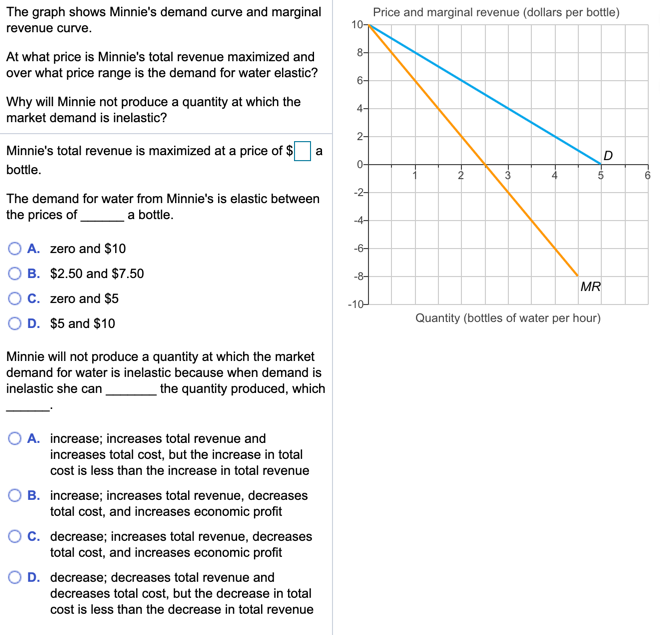Price and marginal revenue (dollars per bottle) The graph shows Minnie's demand curve and marginal revenue curve. At what price is Minnie's total revenue maximized and over what price range is the demand for water elastic? Why will Minnie not produce a quantity at which the market demand is inelastic? a Minnie's total revenue is maximized at a price of \$ bottle. 56 The demand for water from Minnie's is elastic between the prices of a bottle. O A. zero...

• ### A monopoly faces the demand curve P= 11 -0.5Q, where P is measured in dollars per...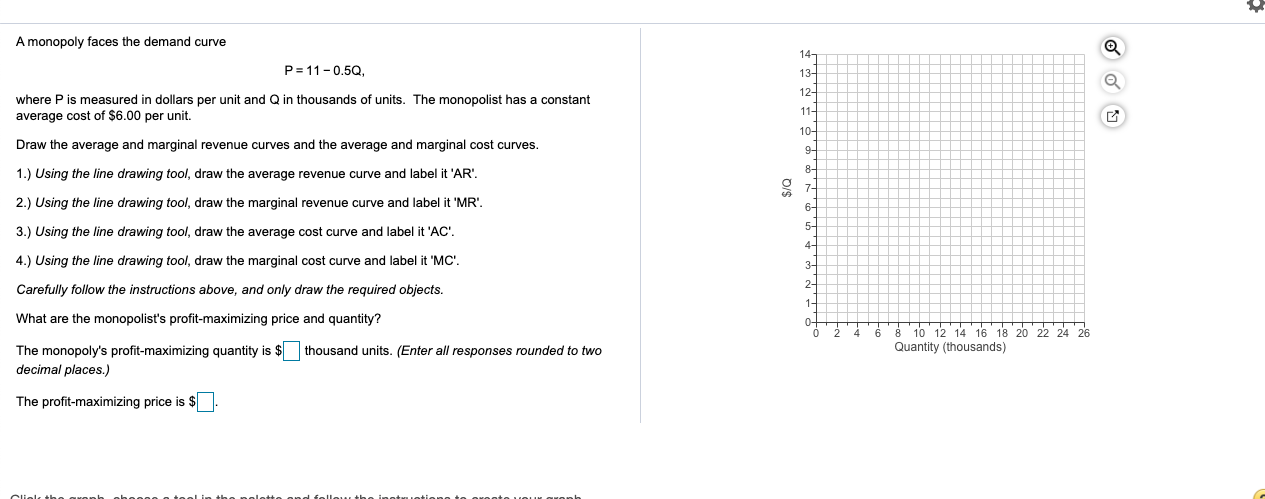A monopoly faces the demand curve P= 11 -0.5Q, where P is measured in dollars per unit and Q in thousands of units. The monopolist has a constant average cost of \$6.00 per unit. Draw the average and marginal revenue curves and the average and marginal cost curves. 1.) Using the line drawing tool, draw the average revenue curve and label it 'AR'. \$/Q 2.) Using the line drawing tool, draw the marginal revenue curve and label it 'MR'. 3.)...